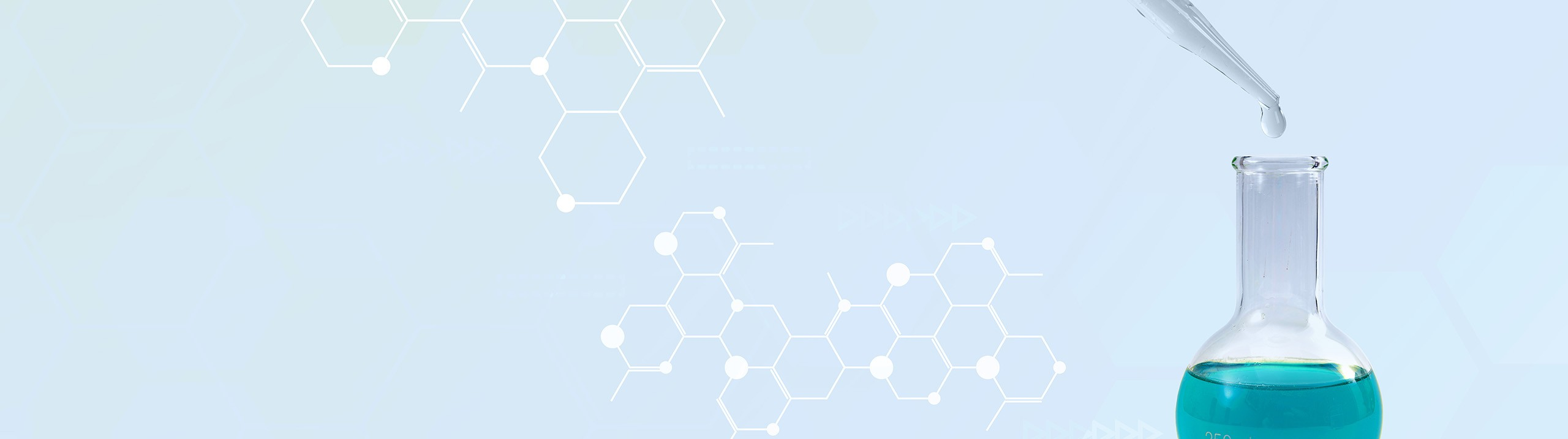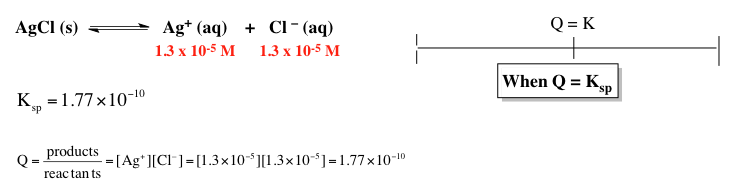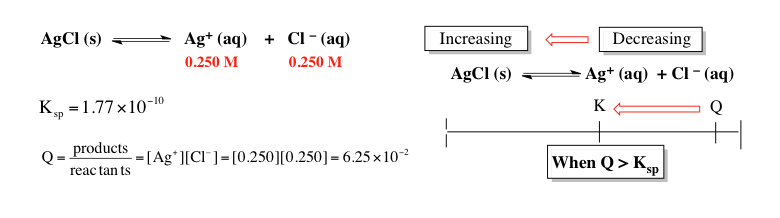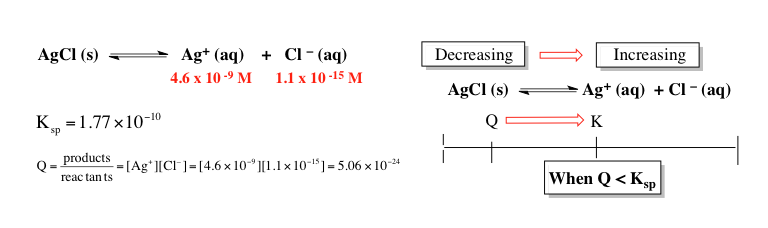## Analytical Chemistry

Learn the toughest concepts covered in your Analytical Chemistry class with step-by-step video tutorials and practice problems.

6. Chemical Equilibrium

# Solubilty Product Constant

The solubility product constant represents the equilibrium value for an ionic solid and its ions in a given solution.

Solubility Product Constant
1
concept

## Solubility Product Constant1m
Play a video:
So here we're gonna say that saul ability represents the maximum amount of solute that could successfully dissolve in a solvent. Remember when a saw you successfully dissolved within a solvent, we create a homogeneous mixture known as a solution. Now we're gonna say associated with any solid and the ionic solid is a Ks. P. Value. This K. S. P. Value is our solid ability product constant. We're gonna say here, the larger your sociability product constant than the more soluble an ionic solid is in a solvent. So the more of it breaks up. And then we're gonna say here, the smaller the psy ability product constant and the less soluble an ionic compound becomes within a solvent. So just remember if we're looking at the suitability of any ionic compound associated with it is a Ks. P. Value. Now that we've gotten that out of the way, click onto the next video and see how we look at example one. When we're asked to figure out what are some liability, product constant is for a hypothetical ionic compound when given its molars liability
2
example

## Solubility Product Constant4m
Play a video:
So here we're told we have a hypothetical compound with the formula mx three and it has a solar cell viability of 30.562 molars. Were asked, what is the value of the cell viability product constant for this hypothetical ionic compound. Now with K. S. P. Here we're dealing with our ionic compound. It's gonna break up into its ions. The metal Which is M breaks off this little three here came from the metal so its original charge was three plus. Remember, ions in solution are Aquarius plus there are three of the X. Negative ion. So here we have our equilibrium equation with K. S. P. We're talking about how it forms an equilibrium and with equilibrium will set up an ice chart. So we have initial change equilibrium. Remember we ignore solids and liquids within an ice chart? They're both breaking the whole thing is breaking up in pure water so initially our ions are zero and zero but their products or their being formed. So the change is going to be plus X. For this, this is a three here. So this is plus three X. Bring down everything. So plus X plus three X. Now K. S. P. Is an equilibrium constant. And like all of the equilibrium constants, it equals products. Overreact ints. But here, when we're dealing with a saturated solution, we're gonna say here that we have our ionic compound is a solid so it's going to be ignored. Remember we ignore solids and liquids. So que sp will just equal products. So in this case it will equal M three plus times X minus the three here makes this three X. But it also cubes. It it becomes the power we're gonna plug in. What we know at equilibrium for each one of these ions. So at equilibrium M three plus is X. And X minus is three X. So plug it in. So X times three X. Which is cute. So that's gonna be X times three cubed is three times three times three which is 27 then this is X cubed. Multiplied together, gives us 27 X. To the fourth. So that's what K. S. P. Equals. Now realize here when they give us the saw ability of the ionic compound that saw ability itself represents X. So all we're gonna do now is plug that value in for X. So we'll write it over here. So K. S. P. Equals 27 X. To the fourth. So that's 27 times 270.562. Which is gonna be to the fourth. What you're gonna do here is take that number to the fourth then multiply by 27. So 27 is gonna multiply 9.9754 times 10 to the negative 10 Multiply that by 27. So that comes out to 2.69 times 10 to the -8. And like other equilibrium constant K. S. P. Has no values. So it's just that number which gives us option D. So just realize here when they're telling us the Mueller saw liability, they're giving us what X equals for the entire ionic compound. Using that is key to determine your K. S. P. Now that you've seen example one take a look and see if you can do example to hear. We're asked to figure out the highest moller saw the ability of each ionic compound given. So here we have to determine what the X. Variable will be from our working example one, you just have to look at things backwards, moving in the opposite direction to help us to isolate X for each situation. And from that you'll be able to see which one has the highest X. Value and therefore the highest moller saw ability.
3
example

## Solubility Product Constant8m
Play a video:
Solubility Product Constant Calculations
4
example

## Solubility Product Constant Calculations 17m
Play a video:
5
example

## Solubility Product Constant Calculations 14m
Play a video:
So here it says find the ph of a saturated solution of aluminum hydroxide. We're told that the K. S. P. Of aluminum hydroxide is 1.9 times 10 to the negative 10. Alright so we know that we're dealing with K. S. P. So we're dealing with this ionic solid. And we're discussing how it breaks up into ions when thrown into solution. So breaks up into aluminum ion Plus three hydroxide ions. Hopefully by following the example up above you're able to get this far in terms of this problem. The steps are very similar to what we saw up above. Except now we're being asked to find ph now here we're gonna have initial change equilibrium again. Remember solids and liquids are ignored within our ice chart. We're gonna say here initially this is zero and this is zero. This is plus X. Just like up above we have +30. H minus is here. So this is three X. So plus X plus three X. K. S. P equals products. Overreact ints. But we ignore the reactant because it's a solid. So it equals aluminum times O. H minus. It's gonna be cubed because of the three as a coefficient K. S. P. Is 1.9 times 10 to the negative 10 X Times three X. Which will be cubed. So just like up above we have 27 X. To the fourth again Equals 1.9 times 10 to the negative 10, Divide both sides here by 27. And we'll get what X. Fourth X. To the fourth equals. So X. To the fourth equals 7.3704 times 10 to the negative 12. Again like up above we're gonna take the fourth root. Or you could do it to the 1/4 power to find X. X. equals 1.629 times 10 to the -3 Moeller. This represents the concentration of my entire ionic compound. But remember we're looking for ph here. Now in our equation we don't have a choice 30 plus anywhere. But what we have instead is oh H minus. If I know H minus is concentration I can find my P. O. H. And from there I can find ph so let's figure out what the concentration of O. H minus is at equilibrium. O. H minus equals three X. So h minus equals three X. So that's three times 1.629 times 10 to the minus three. So that's gonna come out to 4.886 times 10 to the -3 moller. Now that we have the polarity, we can figure out what my ph is. So first we're gonna take the negative log of this number to find P. O. H. Which is the negative log of O. H minus. So that comes out to 2.31. Now that we have P. O. H. We know ph because ph equals 14 minus P. O. H. So that comes out to 11.69 as my ph for this solution. So if you are able to follow along with the example up above this question was similar up to a point once we found the concentration of O H minus in this example, we didn't need to figure out its grams or it's moles. Instead, we'd use those concentrations that we found to figure out our p O H from p O. H, transforming into p H. And we have our answer at the end. So, following all the normal conventions that we saw up above, we see that our ph is equal to 11.69.
6
example

## Solubility Product Constant Calculations3m
Play a video:
So here it says calculate the molar saw the ability of iron to carbonate in a solution of 0.167 moller of sodium carbonate here we're told that the K. S. P. For iron to carbonate is 2.1 times 10 to the negative 11. Alright, so we're dealing with iron to carbonate and were given its K. S. P. Which means that we know we're dealing with the solid form of it. We look at how it breaks up into ions. Now realize up to this point we're normally seeing these ionic compounds breaking up in pure water. But in this case it's breaking up in a solution that is comprised of sodium carbonate, realize sodium carbonate breaks up into sodium ion and carbonate ion. And the carbonate ion is what's important because that carbonate ion is the same carbonate ion here and we're gonna say here because we're breaking up in a solution that already possesses carbonate ion, we're dealing with the common ion effect. So with the common iron effect, this is a solid organ, ignore. There's no common iron of this, this zero with the common iron effect because this carbonate, which the solution is comprised of, is the same as this ion here. The initial concentration will be .00167. Then they're both products or they're both being made. So this is plus X plus X plus X. And then 0.167 plus X. Now with K. S. P. Remember we're concerned with the product because the reaction here is a solid, it's ignored. K. S. P. Equals F. e. two plus times C. 0. 3 2 -. So here K. S. P. Is 2.1 times 10 to the negative 11 equals X. Times Now with K. S. P. We're gonna say because K. S. P. Values are normally so low for the sake ability of these ionic compounds. That means that we can ignore our X. Variable as long as there is a number That is in front of it that precedes it. So we're gonna have .00167 here we're looking for the moller saw the ability of iron to carbonate. So that just means we're looking for X. So divide both sides here by .00167. And when we do that that's gonna give us our X. Variable which is our molars liability for the entire ionic compound that comes out to 1.26 times 10 to the negative eight moller. So remember with the common i in effect we're no longer breaking up our ionic compound in pure water. We're breaking up in a solution that possesses a common ion to one of the ions in are ionic compound. So taking this to heart, attempt to do practice question one. If you get stuck don't worry just come back and take a look at how I approach that same exact practice question and how we get the answer that we're looking for
7
Problem

What is the molar solubility of Fe(OH) 3 (s) in a solution that is buffered at pH 3.50 at 25°C? The Ksp of Fe(OH)3 is 6.3 x 10–38 at 25°C.

The Reaction Quotient & Precipitation
8
concept

## Reaction Quotient & Precipitation2m
Play a video:
So here we're talking about the reaction quotient Q. We're gonna say here that the reaction quotient Q. Is used to determine if our chemical reaction is at equilibrium when we compare it to K. Or if we produce a precipitator solid and that's when we compare it to K. S. P. Remember regular Ks. Your equilibrium constant. K. Sp is also an equilibrium constant, but K. S. P. Is an equilibrium constant for ionic solids. Now here we're gonna say if the reaction quotient Q is equal to the equilibrium constant K. Then our reaction is at equilibrium. For example, here we have silver chloride as our solid, it breaks up into silver ion and chloride ion here were given the concentrations initially of silver and chloride as 1.3 times 10 to negative five molar. Now Q is just like K. It equals products. Overreact ints. And just like our equilibrium constant, K. S. P. We ignore solids and liquids, so Q. Equals just products. So it equals silver ion times chloride ion plugging those values in gives us our Q. Value here are Q. Is 1.77 times 10 to negative 10. Just like K. S. P. Is 1.77 times 10 to the negative 10. They're equal to one another. So we can say because Q and K equal each other here we're at equilibrium in terms of shifting of our chemical reaction, there would be no shifting of our chemical reaction because our chemical reaction is at equilibrium. Now later on when we look up new values for Q. If Q happens to be different from K, this will determine the direction our chemical reaction will shift in order to re attain equilibrium conditions. The shifting can have an effect on the amount of reactant or product that's used or created. Now, click onto the next video and see what happens when our Q value is different from r. K value or k. S. P value.
9
concept

## Reaction Quotient & Precipitation4m
Play a video:
So here we continue our discussion of the comparison of the reaction quotient Q to any type of equilibrium constant, including K. S. P. Now in these next two scenarios, they will not be equal to one another because Q and K will not be equal to one another. This will cause my chemical reaction to shift either in the four directions or the reverse direction to reestablish equilibrium. Now, in this first example here were given new concentrations for ions. So here we have 40.250 molar for each one. And when we plug them into the equilibrium expression for Q, We can see that the value of Q comes out to 6.25 times 10 to the -2. In this case we're gonna see that Q. Is bigger than our K. S. P. Which is still the same value. Remember anyway this K. S. P. Or any type of K would change as if there was a change in temperature. So R K. S. P is remaining the same because temperature is being held constant. But the concentration of my ions are changing which will have a direct impact on the value of Q. So here Q. Is larger than K. S. P. So if Q. Is larger than K. S. P. Or K in general, then our reaction will shift. So on terms of our number line Q will always shift to wherever K. Is. So here Q will shift this way to get the K. And if it shifts that way to get to K, then our chemical reaction will also shift as a whole to get to equilibrium. Remember wherever you shift that direction will cause an increase. So here we're shifting towards the react inside. So the reacting side would be increasing. If the reacting side is increasing, that means our product side is decreasing. So we're gonna say if Q. Is larger than K, then our chemical reaction will shift to the reactant side in this case because we're shifting towards the reacting which happens to be a solid. That means that the precipitate will increase. The amount of solid will increase. Now here, in this case we have new concentrations yet again for the ions again temperatures not being affected. So our equilibrium constant K. S. P remains the same. So in this case we have these new concentrations which gives us a new Q value which is 5.0 times 10. 5.6 times 10 to the negative 24. We can see now that that value is less than K. S. P. So if Q. Is smaller than K. S. P. Or K in general. So now he was no longer here. Instead you will be over here, it's gonna be smaller. So we're gonna have to shift this way. Now we're gonna shift that way in terms of the number line. And in terms of chemical reaction, remember wherever we're heading towards that side is increasing. So this side would increase this side here would decrease. So our chemical reaction will shift to the product side. So that means that the cell ability of my ionic compound is increasing because I'm making more ions and the amount of precipitator solid will decrease. So just remember Q. Our reaction quotient is just used as a way of determining. Are we at equilibrium When Q equals K. S. P. We're at equilibrium. We will say that our chemical reaction or actually our solution is a saturated solution with no real dominant movement to the reacting side of the product side. Where at equilibrium everything is balanced. So we represent a saturated solution. Then if our Q happens to be larger than R. K. S. P. That means we have an excess of ion. So that's why the reaction is shifting in the reverse direction towards the reactant to recreate some more precipitate. We'd say that this type of process happens within a super saturated solution. And then finally when Q. Is less than K. Sp, we can continue to break down our ionic solid to produce more ions. So when Q. Is less than K. S. P. We'd say that we are an unsaturated solution now that we've explored the connection between our reaction question Q. And K. S. P. Will be more prepared when we take a look at questions dealing with more calculation based questions on the formation or lack thereof, of precipitates

When Q is equal to Ksp the solution is saturated and there is no shifting in the equilibrium position.When Q is greater than Ksp the solution is supersaturated and the reaction shifts in reverse to form more precipitate.When Q is less than Ksp the solution is unsaturated and the reaction shifts forward to form more ions.The Reaction Quotient & Precipitation Calculations
10
example

## Reaction Quotient & Precipitation Calculations 17m
Play a video: